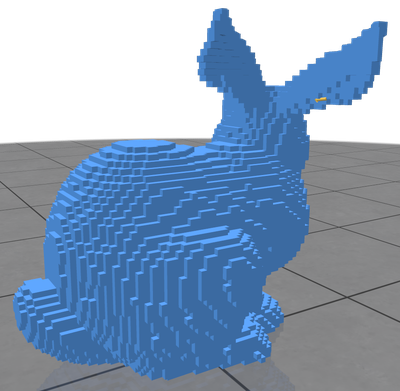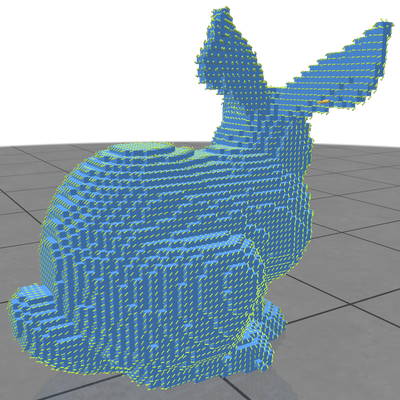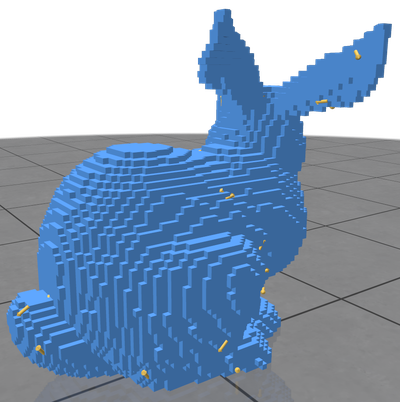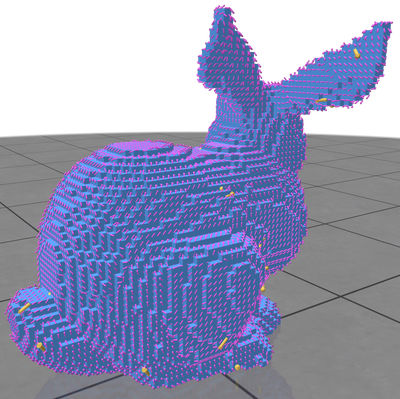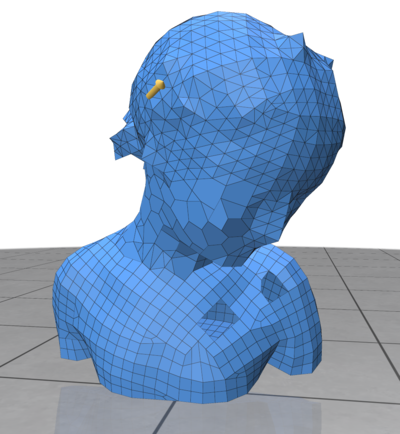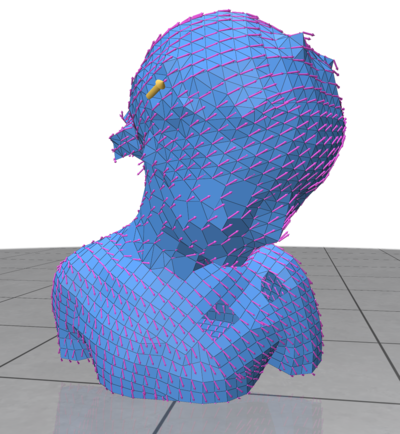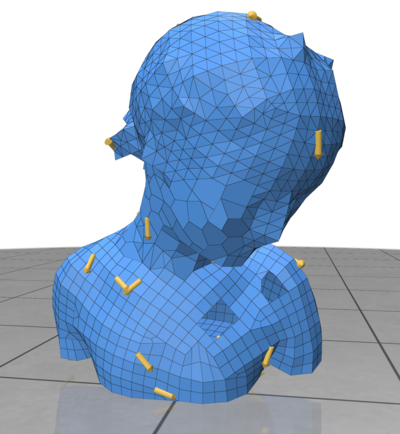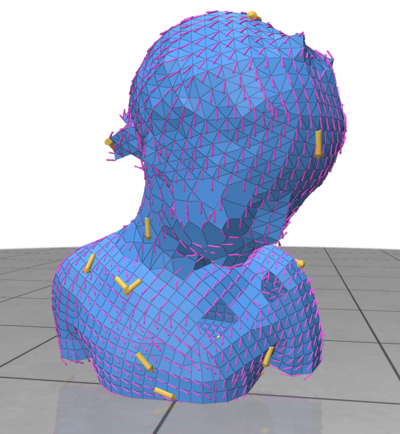DGtal  1.4.beta
Vector Heat Method using discrete polygonal calculus

Author(s) of this documentation:
Baptiste GENEST
Since
1.3

Part of package DEC package.

In this documentation page, we focus on an implementation of the "Vector Heat method" (). The main objective is to highlight the use of differential operators from Discrete differential calculus on polygonal surfaces to solve elementary PDEs on intrinsic vector fields.

Use example can be find at exampleVectorHeatMethod.cpp example file.

Warning
The implementation heavily relies on implicit operators with many Eigen based small matrix constructions, which has a huge overhead in Debug mode. Please consider to build the examples in Release (e.g. CMAKE_BUILD_TYPE variable) for high performance on large geometrical objects.

# The main algorithm

The algorithm consists in solving three linear systems (see  for details):

• Given vectors sources $$\phi$$ at a mesh vertices, we first solve a vector heat diffusion problem: Integrate the heat flow $$u$$ such that $$\Delta^{\nabla} u = \frac{\partial u}{\partial t}$$ using a single step backward Euler step: $$(Id - t\Delta^{\nabla}) u_t = \phi$$. That step diffuses vector directions correctly using Levi-Civita connection but not vector magnitudes hence we then solve the two following (scalar) diffusion systems:
• $$(Id - t\Delta) m_t = ||\phi||$$
• $$(Id - t\Delta) \delta_t = 1_{\phi \neq 0}$$
• To finally evaluate the vector field : $$X_t = \frac{u_t}{||u_t||}\frac{m_t}{\delta_t}$$

The computation involves discrete differential operator definitions (Connection Laplace and standard Laplace-Beltrami) as well as linear solvers on sparse matrices. We do not go into the details of the discretization, please have a look to the paper if interested.

# The interface

The class VectorsInHeat contains the implementation of the Vector Heat method. It relies on the PolygonalCalculus class for the differential operators (Discrete differential calculus on polygonal surfaces).

First, we need to instantiate the VectorsInHeat class from an instance of PolygonalCalculus:

typedef PolygonalCalculus<SurfaceMesh<RealPoint,RealPoint>> Calculus;
Calculus aCalculus( mesh );
VectorsInHeat<Calculus> heat( aCalculus );

Then, we can prefactorized the solvers for a given a timestep $$dt$$:

heat.init(dt);
Note
For a discussion on the timestep please refer to . For short, the authors suggest a timestep in $$dt=m\cdot h^2$$ for some constant $$m$$ and $$h$$ being the mean spacing between adjacent vertices.

Once prefactorized, we can add as many sources as we want using the method:

heat.addSource( aVertexIndex, 3Dvector ) //the vector will be projected on the vertex tangent plane
...
Note
the vertex index corresponds to the indexing system of the underlying SurfaceMesh instance.

The resulting interpolating vector field is obtained by:

auto u = heat.compute();

# Examples

From exampleVectorHeatMethod.cpp code on a digital surface and a generic polygonal one.

Input Result Multiple Inputs Result